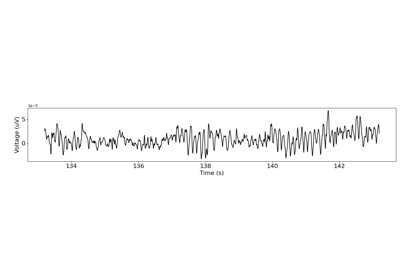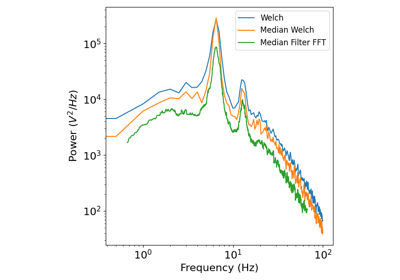# neurodsp.spectral.compute_spectrum¶

neurodsp.spectral.compute_spectrum(sig, fs, method='welch', avg_type='mean', **kwargs)[source]

Compute the power spectral density of a time series.

Parameters
sig1d or 2d array

Time series.

fsfloat

Sampling rate, in Hz.

method{‘welch’, ‘wavelet’, ‘medfilt’}, optional

Method to use to estimate the power spectrum.

avg_type{‘mean’, ‘median’}, optional

If relevant, the method to average across windows to create the spectrum.

**kwargs

Keyword arguments to pass through to the function that calculates the spectrum.

Returns
freqs1d array

Frequencies at which the measure was calculated.

spectrum1d or 2d array

Power spectral density.

Examples

Compute the power spectrum of a simulated time series:

>>> from neurodsp.sim import sim_combined
>>> sig = sim_combined(n_seconds=10, fs=500,
...                    components={'sim_powerlaw': {}, 'sim_oscillation' : {'freq': 10}})
>>> freqs, spectrum = compute_spectrum(sig, fs=500)


## Examples using neurodsp.spectral.compute_spectrum¶